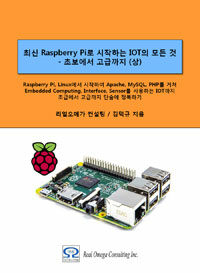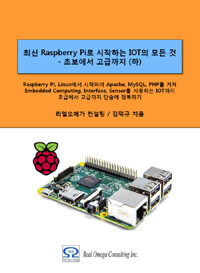# Raspberry Pi_Eng_23.5.6 Learning Python Using Simple Example

#### Published Book on AmazonAll of IOT Starting with the Latest Raspberry Pi from Beginner to Advanced – Volume 1All of IOT Starting with the Latest Raspberry Pi from Beginner to Advanced – Volume 2

#### 출판된 한글판 도서최신 라즈베리파이(Raspberry Pi)로 시작하는 사물인터넷(IOT)의 모든 것 – 초보에서 고급까지 (상)최신 라즈베리파이(Raspberry Pi)로 시작하는 사물인터넷(IOT)의 모든 것 – 초보에서 고급까지 (하)

### 23.5.6Learning Python Using Simple Example

Number-matching game example

This program is an interactive, number-matching game. It allows users to match number from 1 to 99.

Here "randint" function is used to get a random number. This program will continue to run using "while" loop until the user match the number.

 import random n = random.randint(1, 99) guess = int(raw_input("Enter an integer from 1 to 99: ")) while n != "guess":     print     if guess < n:         print "guess is low"         guess = int(raw_input("Enter an integer from 1 to 99: "))     elif guess > n:         print "guess is high"         guess = int(raw_input("Enter an integer from 1 to 99: "))     else:         print "you guessed it!"         break     print

Average calculation example

The following example is a program that takes three input values and calculates an average value. Integer number must be entered.

 # Get three test score round1 = int(raw_input("Enter score for round 1: ")) round2 = int(raw_input("Enter score for round 2: ")) round3 = int(raw_input("Enter score for round 3: "))    # Calculate the average average = (round1 + round2 + round3) / 3   # Print out the test score print "the average score is: ", average